# Show or Hide Formula Bar

This post will guide you how to display a missing formula bar in Excel. How to hide the formula bar in Excel. How to expand the formula bar so that a long formula can be displayed completely.

The Excel Formula bar is a toolbar at the top of the Excel worksheet window, and you can use it to type the excel formula and run it to apply this formula on a specific cell.

## Show or Hide Excel Formula Bar

The Formula bar is a very useful toolbar in your worksheets. And if the formula bar is missing in your current workbook, you can refer to the following steps to unhide it.

#1 Go to VIEW tab, select the Formula Bar checkbox under Show group. If you want to hide the formula bar, just unchecking the Formula bar option.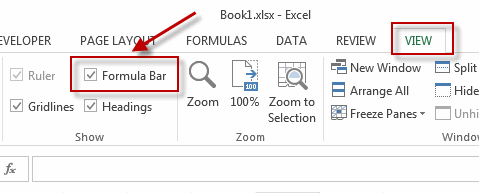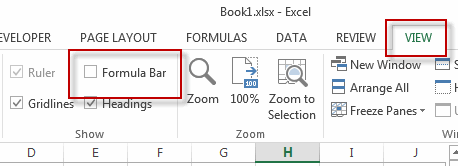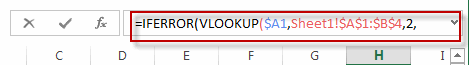Note:

You can also unhide the formula bar with Excel Options feature, just do the following steps:

#1 Go to File tab, click Options menu from the pop-up menu list. The Excel Options dialog will appear.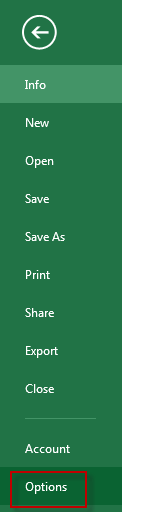#2 click Advanced in the left pane.

#3 select the Show formula bar in the Display section.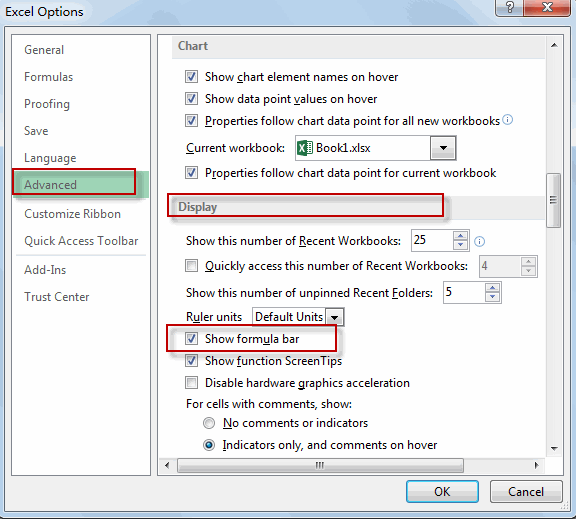## Expand Excel Formula Bar

If your formula is too long and it will be not displayed completely, at this moment, you can expand the formula bar. You just need to hover the mouse near the bottom of the formula bar, then drag the mouse down until the formula bar gets big enough to fit the entire formula in.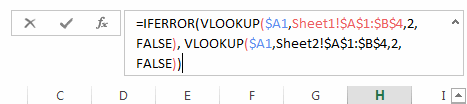Note: you can also use the shortcut Ctrl + Shift +U to expand the formula bar in Excel.